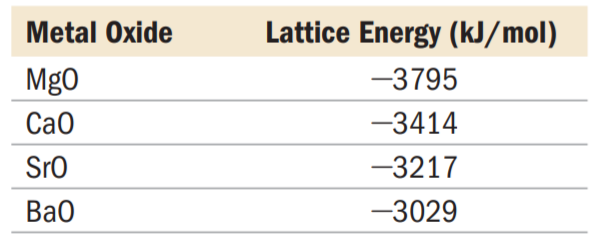×
Get Full Access to Chemistry: A Molecular Approach - 3 Edition - Chapter 9 - Problem 43e
Get Full Access to Chemistry: A Molecular Approach - 3 Edition - Chapter 9 - Problem 43e

×

# Explain the trend in the lattice energies of the alkalineISBN: 9780321809247 1

## Solution for problem 43E Chapter 9

Chemistry: A Molecular Approach | 3rd Edition

• Textbook Solutions
• 2901 Step-by-step solutions solved by professors and subject experts
• Get 24/7 help from StudySoup virtual teaching assistantsChemistry: A Molecular Approach | 3rd Edition

4 5 1 333 Reviews
12
2
Problem 43E

Explain the trend in the lattice energies of the alkaline earth metal oxides.Step-by-Step Solution:

Solution 43 E Step 1 of 1 We are considering oxides of elements belonging to the same group (II A). We know that the ionic radii increases while we pass from the top to the bottom of a group (it happens the same if we consider the atomic radii). Moreover, we know that lattice energy depends on the size of anions and cations that are in the compound. In fact there’s a reverse proportionality between dimensions and energy: the bigger the dimension, the smaller the energy and vice-versa. Since Mg is the atom with smaller dimensions, we can expect that MgO has the highest lattice energy for this group of oxides. Since Ba is the atom with the bigger dimension, we can expect the lowest lattice energy for this group of oxides. Obviously Ca and Sr have intermediate dimensions respect to Mg an Ba, thus we can even expect intermediate values of lattice energy, but CaO has the more negative value than SrO because Ca is smaller than Sr.

Step 2 of 1

##### ISBN: 9780321809247

This full solution covers the following key subjects: alkaline, EARTH, energies, explain, lattice. This expansive textbook survival guide covers 82 chapters, and 9454 solutions. This textbook survival guide was created for the textbook: Chemistry: A Molecular Approach, edition: 3. Since the solution to 43E from 9 chapter was answered, more than 1335 students have viewed the full step-by-step answer. The answer to “?Explain the trend in the lattice energies of the alkaline earth metal oxides.” is broken down into a number of easy to follow steps, and 13 words. The full step-by-step solution to problem: 43E from chapter: 9 was answered by , our top Chemistry solution expert on 02/22/17, 04:35PM. Chemistry: A Molecular Approach was written by and is associated to the ISBN: 9780321809247.

Unlock Textbook Solution